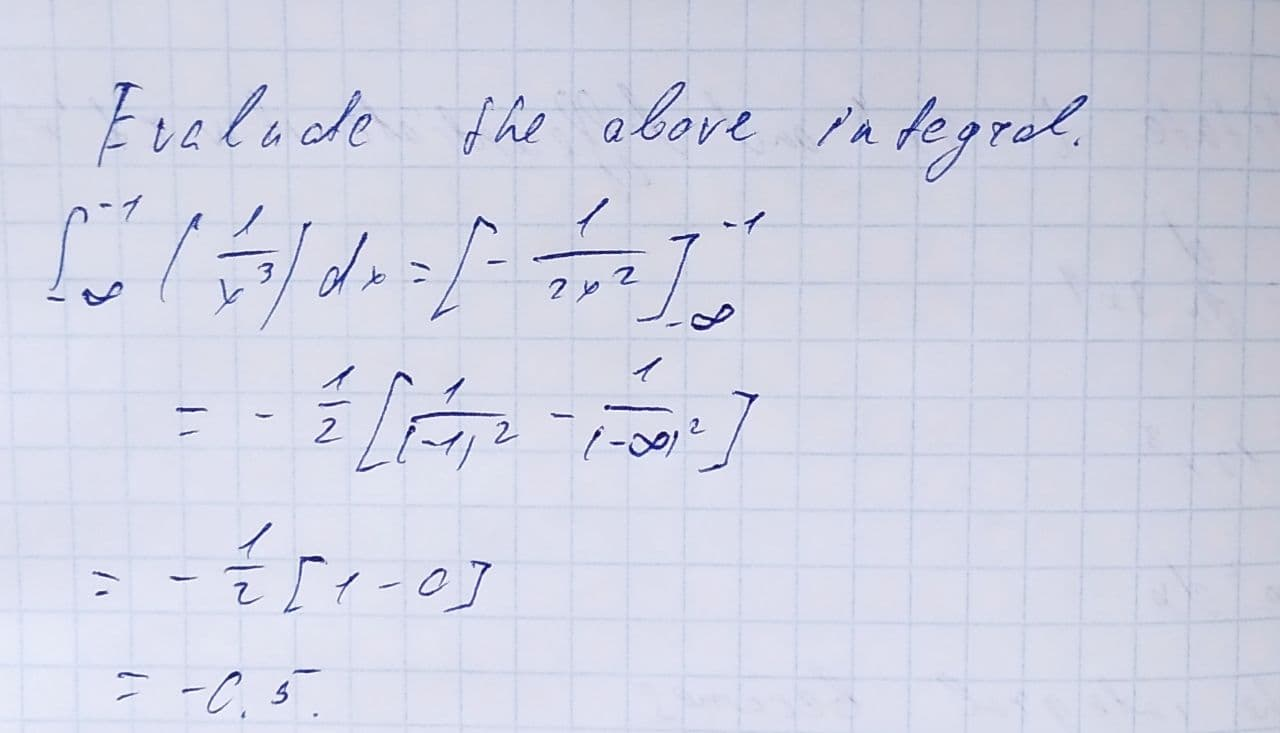Evaluate the following integrals or stat that they diverge. \int_{-\infty}^{-1}\frac{dx}{x^{3}}Jaya Legge 2021-08-11 Answered
Evaluate the following integrals or stat that they diverge.
$$\displaystyle{\int_{{-\infty}}^{{-{1}}}}{\frac{{{\left.{d}{x}\right.}}}{{{x}^{{{3}}}}}}$$

• Questions are typically answered in as fast as 30 minutes

Solve your problem for the price of one coffee

• Math expert for every subject
• Pay only if we can solve itMacsen Nixon
Our aim is to evaluate the integral given below